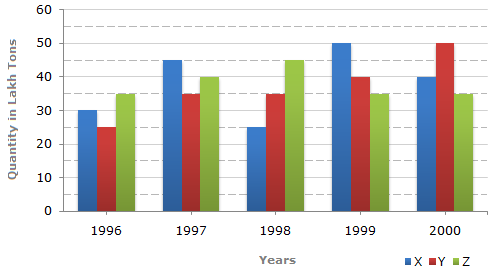# Data Interpretation - Bar Charts - Discussion

The bar graph given below shows the data of the production of paper (in lakh tonnes) by three different companies X, Y and Z over the years.

Production of Paper (in lakh tonnes) by Three Companies X, Y and Z over the Years.2.

What is the ratio of the average production of Company X in the period 1998-2000 to the average production of Company Y in the same period?

 [A]. 1:1 [B]. 15:17 [C]. 23:25 [D]. 27:29

Explanation:

Average production of Company X in the period 1998-2000

 =1 x (25 + 50 + 40)=115lakh tons. 3 3

Average production of Company Y in the period 1998-2000

 =1 x (35 + 40 + 50)=125lakh tons. 3 3Required ratio =1153
= 115 = 23 .1253
125 25

 Maria said: (Jul 8, 2016) Why did they not calculate the average for fraction part? (115/3) / (125/3).

 Sandeep said: (Nov 9, 2016) Can anyone explain the step (115/3)/(125/3) = 23/25?

 Kamalpreet Kaur said: (Mar 24, 2018) How this answer 23/25? because after average the answer is different.